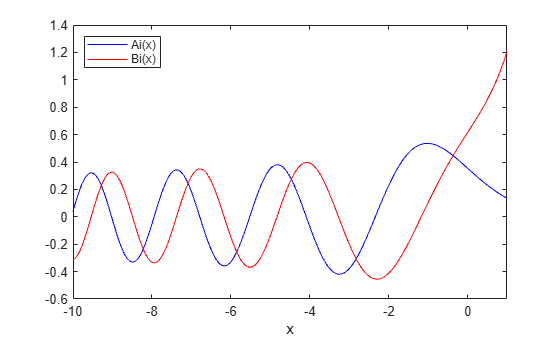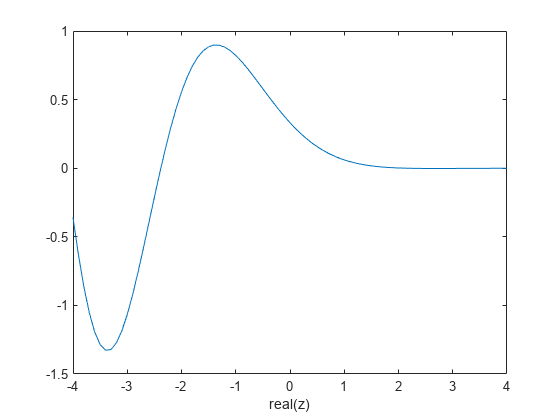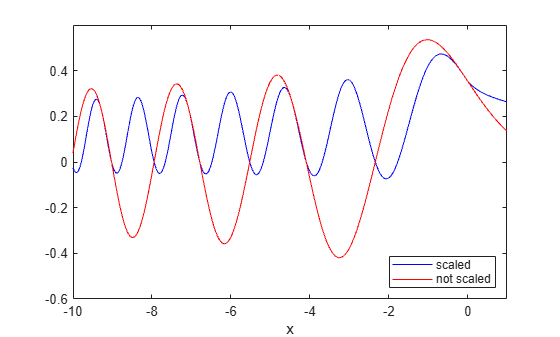airy

Airy Functions

Description

example

W = airy(Z) returns the Airy function, Ai(Z), for each element of Z.

example

W = airy(k,Z) returns any of four different Airy functions, depending on the value of k, such as the Airy function of the second kind or the first derivative of an Airy function.

example

W = airy(k,Z,scale) scales the resulting Airy function. airy applies a specific scaling function to W depending on your choice of k and scale.

Examples

collapse all

Define x.

x = -10:0.01:1;

Calculate Ai(x)

ai = airy(x);

Calculate Bi(x) using $k=2$.

bi = airy(2,x);

Plot both results together on the same axes.

figure
plot(x,ai,'-b',x,bi,'-r')
axis([-10 1 -0.6 1.4])
xlabel('x')
legend('Ai(x)','Bi(x)','Location','NorthWest')Compute the Airy function at a slice through the complex plane at $x+i$.

Take a slice through the complex plane.

x = -4:0.1:4;
z = x+1i;

Calculate Ai(z).

w = airy(z);

Plot the real part of the result.

figure
plot(x, real(w))
axis([-4 4 -1.5 1])
xlabel('real(z)')Define x.

x = -10:0.01:1;

Calculate the scaled and unscaled Airy function.

scaledAi = airy(0,x,1);
noscaleAi =  airy(0,x,0);

Plot the real part of each result.

rscaled = real(scaledAi);
rnoscale = real(noscaleAi);
figure
plot(x,rscaled,'-b',x,rnoscale,'-r')
axis([-10 1 -0.60 0.60])
xlabel('x')
legend('scaled','not scaled','Location','SouthEast')Input Arguments

collapse all

System variable, specified as a real or complex vector, matrix, or N-D array.

Data Types: single | double
Complex Number Support: Yes

Type of Airy function, specified as one of four values.

k

Returns

0

Airy function,$Ai\left(Z\right)$, which is the same as airy(Z).

1

First derivative of Airy function, $A{i}^{\prime }\left(Z\right)$.

2

Airy function of the second kind,$Bi\left(Z\right)$

3

First derivative of Airy function of the second kind,$B{i}^{\prime }\left(Z\right)$

Data Types: single | double

Scaling option, specified as 0 or 1. Use scale = 1 to enable the scaling of Z. The values you specify for k and scale determine the scaling function airy applies to Z.

scalekScaling applied to output
0Any

None

10 or 1

${e}^{\frac{2}{3}{Z}^{\left(3/2\right)}}$

12 or 3

${e}^{-|\frac{2}{3}Re\left({Z}^{\left(3/2\right)}\right)|}$

Data Types: single | double

Output Arguments

collapse all

Airy function of Z, returned as an array the same size as Z.

collapse all

Airy Functions

The Airy functions form a pair of linearly independent solutions to

$\frac{{d}^{2}W}{d{Z}^{2}}-ZW=0.$

The relationship between the Airy and modified Bessel functions is

$\begin{array}{l}Ai\left(Z\right)=\left[\frac{1}{\pi }\sqrt{\frac{Z}{3}}\right]{K}_{1/3}\left(\zeta \right)\\ Bi\left(Z\right)=\sqrt{\frac{Z}{3}}\text{\hspace{0.17em}}\text{\hspace{0.17em}}\left[{I}_{-1/3}\left(\zeta \right)+{I}_{1/3}\left(\zeta \right)\right],\end{array}$

where

$\zeta =\frac{2}{3}{Z}^{3/2}.$